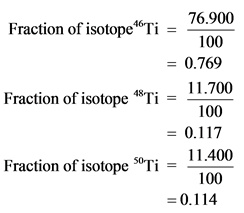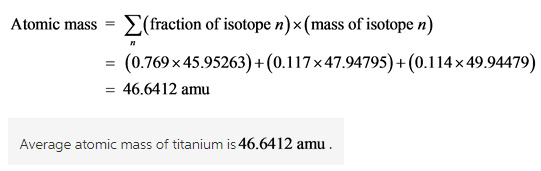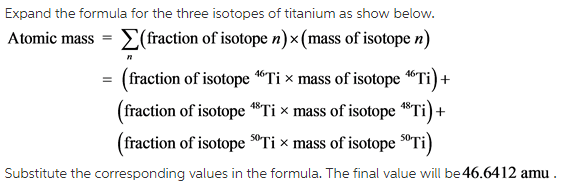# On another planet, the isotopes of titanium have the following natural abundances. Isotope Abundance Mass

On another planet, the isotopes of titanium have the following natural abundances.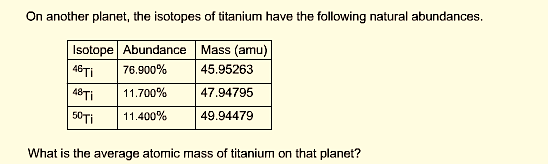Concepts and reason
Average atomic mass of an element is mass of the element that can be calculated by using their abundance and masses.
Calculate the average atomic mass of the element titanium by using the following formula.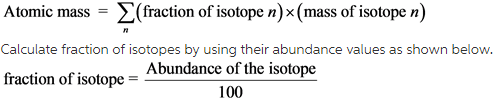Now calculate the fraction for the three different titanium isotopes and substitute these values along with their masses in the above formula.

Fundamentals
Atoms with same atomic number and different mass number are called as isotopes.
Atomic number of the atom is equal to the number of protons; mass number is equal to the sum of protons and neutrons.